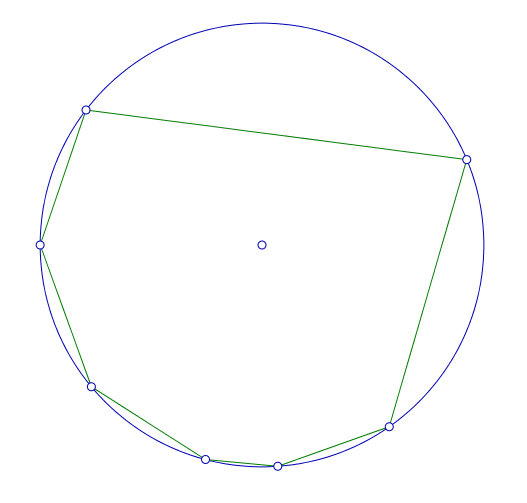# The center lies within ....

Probability Level 5Pick $7$ points at random on the circumference of a unit circle. The probability that the center of the circle lies within the (unique) convex polygon defined by these $7$ random points is $\dfrac{a}{b}$, where $a$ and $b$ are positive coprime integers.

Find $a + b$.

×